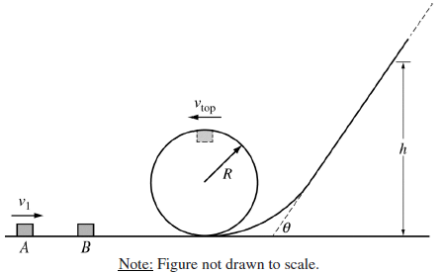# 2016 AP Physics 1物理Algebra-Based真题系列之Practice Exam简答题免费下载

### 真题与答案下载### 真题下载请前往【纯真题】小程序### 2016 AP 物理1模考简答题部分免费下载

#### 部分真题预览：1）Two blocks A and B of equal mass m are on a frictionless track, as shown in the figure above. Block A, initially moving with speed v1 , has a perfectly elastic collision with block B. Block B has a speed v1 immediately after the collision, and then it travels around a circular loop of radius R, where R is much larger than the size of the blocks. The speed of block B at the top of the loop is vtop. Block B then slides up a ramp until it comes momentarily to rest at a height h above the floor.

1. Derive an equation for the height h in terms of m, R, vtop , and g, as appropriate.
Unauthorized
2. Suppose that the speed v1 of block A is doubled. Will the final height of block B be greater than, less than, or equal to 2h ?
____ Greater than ____ Less than 2h ____ Equal to
Briefly explain how you arrived at your answer.3. Blocks A and B (both of mass m) are moved to a different frictionless track, as shown above. Let vA be the minimum initial speed for block A that allows block B to make it over the hump in the track. Block A is then replaced with a larger block, block C, which has mass 2m, as shown below.Block C makes a completely inelastic collision with block B so that both blocks stick together and travel along the track. What is the minimum initial speed vC that block C must have so that the two-block system makes it over the hump? Express your answer in terms of vA .

### 2016 AP 物理1简答题免费下载